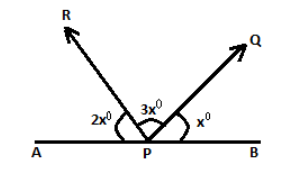In the given figure, $\angle QPB$ isA) $60^\circ$B) $45^\circ$C) $30^\circ$D) $15^\circ$Verified
148.2k+ views
Hint:: To solve this problem, we have to know a basic rule that the sum of all angles on a straight line is ${180^\circ}$. First of all sum up the given angle values (in unknown term) which is equal to ${180^\circ}$and then find the value of $x$ which is also the value of $\angle QPB$.

Complete step by step solution:
All the given angles on a straight line after summing up will be equal to ${180^\circ}$.
So, $2x + 3x + x = {180^\circ}$
On simplifying the above $x$ terms,
$\Rightarrow 6x = {180^\circ}$
Solving for the value of $x$,
$\Rightarrow n = \dfrac{{{{180}^\circ}}}{6} = {30^\circ}$
As per given information in the question, $\angle QPB = {x^\circ} = {30^\circ}$.

$\therefore$ $\angle QPB = {x^\circ} = {30^\circ}$. The correct option is (C).

Note:
First of all, read the question attentively so that you don’t get into any confusion about what is asked for as the ultimate answer. Solve for the unknown term in the linear equation obtained from the given information in the question. Do the calculations properly to ensure that you don’t make a silly mistake even after knowing the procedure to solve it. The only basic thing to be kept in mind is that the sum of all angles on a straight line is ${180^\circ}$.